Math Concepts
What is One to one correspondence?
7.7k views

 1 Introduction 2 One to One Correspondence 3 Invertible Functions 4 How to Tell if a Function is Invertible 5 Bijective Function examples 6 Summary 7 FAQs

## Introduction

Let's take some real life examples based on this so if you can think  about "what inventions are based on one-to-one correspondence", the answer is "everything".I can say that , it's so basic that it's used to describe a lot of functions.For example

Hash tables are a fundamental type of data structure in computer programming that  might (but not necessarily) give one to one correspondence between keys and values for computation . To design good software it's important on some level to understand the concept of a one to one correspondence, as well as how it can fail (and how likely it is to fail in a given situation, what to do when it does fail, etc.).There is a one to one correspondence between certain dots on a train map and actual train stations. There is a 1-to-1 correspondence between times of a day and numbers like 10:55 am. Whenever you count something you establish a 1-to-1 correspondence with a subset of the natural numbers. For instance track numbers on an album correspond to songs.

### What is One to one correspondence?-PDF

This blog will give a description of what one to one correspondence means, how it defines invertible functions and how one can determine whether a function is invertible. Here is a downloadable PDF to explore more.

## One to One Correspondence

One-to-One functions define that each element of one set called Set (A) is mapped with a unique element of another set called Set (B).
Or

It can  be defined as each element of Set A has a unique element on Set B.

In short , let us  take  ‘f’ as a function whose domain is set A. The function is said to be injective if for all x and y in A,

When there is  f(x)=f(y), then x=y

And equally , if x ≠ y, then f(x) ≠ f(y)

Now, Onto functions are basically functions whose range equates to its codomain..

Thus,

It is stated as, if f(x) = f(y)  implies x=y, then f is one-to-one mapped and also if the codomain equates to the range of the function, f is one to one correspondence.

Formally , if “f” is a function which is one to one correspondence, with domain A and range B, then the inverse of function f is given by;
f-1(y) = x ; if and only if f(x) = y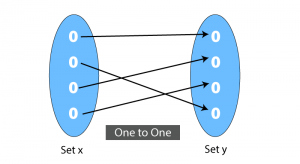A function f : X → Y is said to be one to one correspondence, if the images of unique elements of X under f are unique, i.e., for every x1 , x2 ∈ X, f(x1 ) = f(x2 ) implies x1  = x2 and also range = codomain. Otherwise, we call it a non invertible function or not bijective function.

Therefore we can say, every element of the codomain of one to one correspondence is the image of only one element of its domain.

### One to one correspondence example

Let f : A ----> B be a function. Codomain = {7,9,10,8,4}

The function f is  say is  one to one, if it takes different elements of A into different elements of B.

So then , we say f is one to one

If  f is one-one, if no element in B is associated with more than one element in A.

Also, range is equal to codomain given the function. Thus, this is one to one correspondence or an invertible function.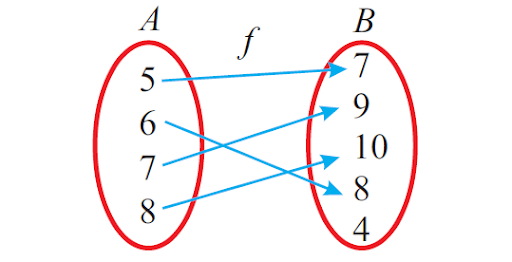### Problem 1 :

Let f : A ----> B. A, B and f are defined as. Codomain is the set { 5,6,7,8}

A  =  {1, 2, 3}

B  =  {5, 6, 7, 8}

f  =  {(1, 5), (2, 8), (3, 6)}

Prove that  whether f is a function. If so, then is it one to one correspondence?

### Solution :

Write the elements of f (ordered pairs) using arrow diagram as shown below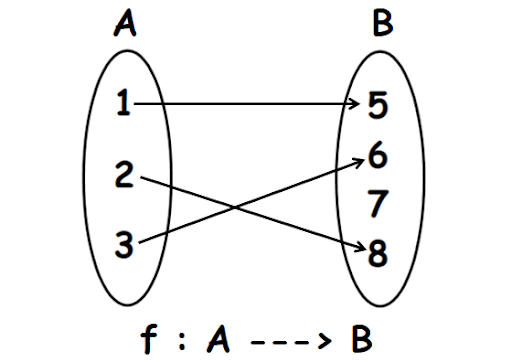In the above arrow diagram, all the elements of A have images in B and every element of A has a unique element .

That is, no element of A has more than one image or unique value..

So, f is a function.

Every element of A has a different value in B.

That is, no two or more elements of A have the same value  in B.

Therefore, f is one to one function.

BUT

Codomain is given as {5,6,7,8} while Range is {5,6,8}. Thus codomain is not equal to range.

Therefore, f is not one to one correspondence.

### Problem 2 :

Let f : X ----> Y. X, Y and f are defined as

X  =  {a, b, c, d}

Y  =  {d, e, f}

f  =  {(a, e), (b, f), (c, e), (d, d)}

Is f one to one correspondence? Explain.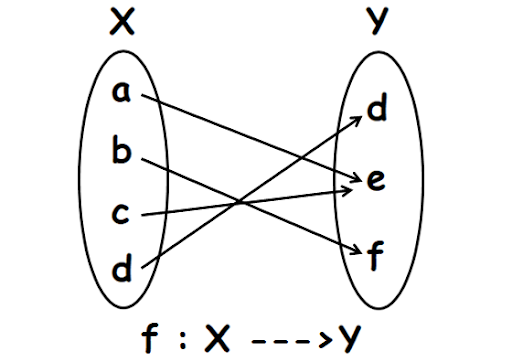In the above arrow diagram, all the elements of X have images in Y and every element of X has a unique element..

That is, no element of X has more than one image.

The elements "a" and "c" in X have the same image "e" in Y.

So, f is not one to one or injective.

Thus, f is not an invertible function or not one to one correspondence.

### Problem 3 :

Let f : A ----> B. A, B and f are defined as

A  =  {1, 2, 3, 4}

B  =  {5, 6, 7, 8}

f  =  {(1, 8), (2, 6), (3, 5), (4, 7)}

Codomain= {5,6,7,8}

Is f invertible or one to one correspondence ? Explain.

### Solution :

Please write the elements of f (ordered pairs) using an arrow diagram as shown below.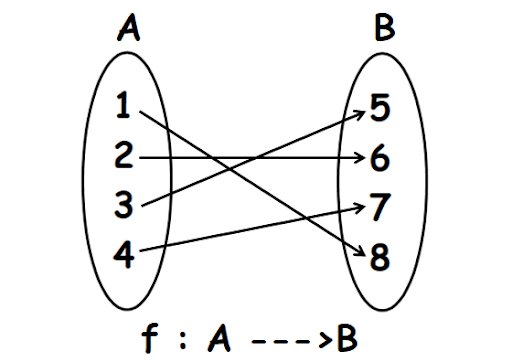In the above  diagram, all the elements of A have images in B and every element of A has a unique image.

That is, no element of A has more than one image.

So, f is a function.

Every element of A has a different element  in B.

That is, no two or more elements of A have the same image in B.

Therefore, f is one to one or injective function.

Also, the codomain is equal to the range which is {5,6,7,8}

Therefore the above mapping is both one to one and onto which makes it one to one correspondence.

## Invertible function

### What is an invertible function?

A function is invertible if we  reverse the order of mapping we  are getting  the input as the new output. In other ways , if a function f whose domain is in set A and image in set B is invertible if f-1 has its domain in B and image in A.
f(x) = y ⇔ f-1 (y) = x.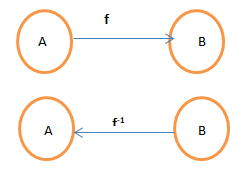Not all functions have an inverse. For a function to be an inverse, each element b∈B must not have more than one a ∈ A. The function would be an Injective function. Also, each and every  element of B must be matched with that of A. The function might be a Surjective function. It is necessary that the function is both one to one and onto to be an invertible function, and vice-versa.

It is good to know  that the composition of a function and its inverse returns the element of the domain.
f-1 o f = f -1 (f(x)) = x

## How to tell if a function is invertible?

Let's take an example of the SSN you have is yours alone: it means that it is unique.

So we can consider the function SSA that associates Americans with their unique Social Security Numbers or SSNs. Now the Social Security Administration (SSA) can take your name and give your SSN; furthermore, if I give them a SSN, they can tell me your name. That is, they can go back and forth between their domain and range. They can go "backwards".So really we can think of two functions here: one, call it  SSA, that has as its domain names, and returns SSN numbers (which are in the range); from that function, we can define another function, call it S S A1 , which has its domain SSN numbers, and which returns names in its range.

• A non-invertible function

Now here's a function that won't work backwards. Consider the function IRS, which takes your name and associates it with the income taxes you paid last year. While the IRS can take your name (and SSN!), and tell how much you paid in taxes (let's say that it was $500), they can't take a dollar amount ($500) and tell your name -- because there might be 100,000 people who paid that amount in taxes. The tax amount is not unique to you, in general.

• a one-to-one function

The first kind of function is called a one-to-one function, and it is invertible -- that is, from it we can create another function which goes backwards, from the range of the original function to the domain. Here's a picture of the situation: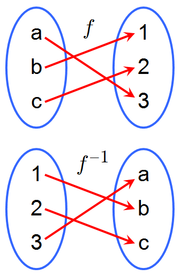Notice the notation: the inverse of f is f-1 , and the domains and ranges of the two are interchanged.

• A graphical test for one-to-one-ness (that is, invertibility)

Graphically, we use the horizontal line test to see if a function is one-to-one: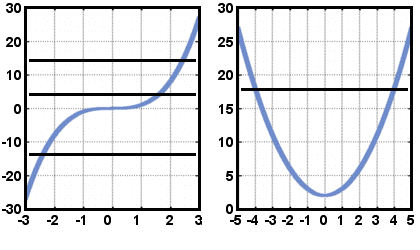Left: one-to-one No horizontal line crosses more than once Right: not one-to-one Here's a horizontal line that crosses more than once

If no horizontal line crosses the function more than once, then the function is one-to-one.
one-to-oneno horizontal line intersects the graph more than once

### Question:

which functions in our function zoo are one-to-one, and hence invertible?

• Inverse function property:

1.:
This saysmapsto, thensendsback to.

2 .:

This saysmapsto, thensendsback to.

• Here's an example. You might know that the square function and the square root function are inverses. That is, if, then. So take an example value of. ThenandThe role ofis played by 2; the role ofis played by 4.

• How to find the inverse of a one-to-one function:
1. Write.
2. Solve this equation for x in terms of y (if possible)
3. Interchange x and y. The resulting equation is.

This is the silly step! Because our favorite independent variable is x, we do this. There's no reason not to leave the inverse asIt will just bother most textbook writers.

In fact, it's better to leave it this way, because it indicates that the domain ofis the range of(and vice versa).

If you understand why I'm making a big deal about this, then you're doing really well. Keep it up! If you don't understand, ask a question.

• Graphing the Inverse of a Function:

It's easy, if you have the graph of: just reflect the graph ofacross the line: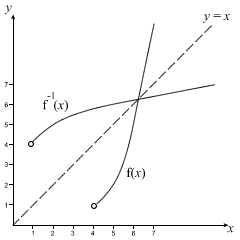Why does this work? Because the inverse functionjust swaps the domain and range of, which means that the x and y-axes are swapped.

• We've already seen examples of invertible functions:

Notice that the example of function composition has invertible functions everywhere: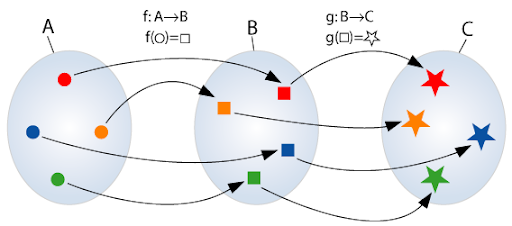From this picture we can conclude that the composition of invertible functions is invertible on its domain.

## Bijective Function examples

1. f(x) = 3x – 5 is a bijective function from R to R.
2. f(x) = x2 is a bijective function from positive R to positive R.
3. f(x) = 1 from R to R

## Summary

Lets now summarize our learning.

### Injective:

An injective function or injection or one-to-one function is a function that preserves uniqueness: it never maps distinct elements of its domain to the same element of its codomain. In other words, every element of the function's codomain is the image of at most one element of its domain.

### Surjective:

A function f from a set X to a set Y is surjective (or onto), or a surjection, if  each and  every element y in Y has a corresponding element x in X such that f(x) = y. (It is not required that x  must be unique; the function f may map one or more elements of X to the same element of Y.)

### Bijective:

A bijective function or one-to-one correspondence is a function between the elements of two sets, where each element of one set is paired with exactly one element of the other set, and each element of the other set is paired with exactly one element of the first set. There are no unpaired elements. In general terms, a bijective function f: X → Y is a one-to-one (injective) and onto (surjective) mapping of a set X to a set Y.

Written by Gargi Shrivastava

## What is one to one correspondence?

Let f : A ----> B be a function. Codomain = {7,9,10,8,4}

The function f is  say is  one to one, if it takes different elements of A into different elements of B.

So then , we say f is one to one

If  f is one-one, if no element in B is associated with more than one element in A.

Also, range is equal to codomain given the function. Thus, this is one to one correspondence or an invertible function.

## Which functions are not said to be one to one?

If a horizontal line can intersect the graph of the  given function, more than one time, then the function is not mapped as one-to-one.

## What is an invertible function?

A function is invertible if we  reverse the order of mapping we  are getting  the input as the new output. In other ways , if a function f whose domain is in set A and image in set B is invertible if f-1 has its domain in B and image in A.
f(x) = y ⇔ f-1 (y) = x.

Related Articles